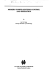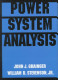### Transmission and Distribution of Electric Power

#### Course Description

History of electrification. Mechanical calculations on overhead lines. Symmetrical components. Inductance and Capacitance. Carson formulea. Transmission Theory. Transmission equations. Ideal lines. Unloaded lines. Short circuit. Optimal transmission. Mathematical models of lines. Pi model, T model, gamma model. Power line transients. Distribution networks. Cable Networks. Cable dimensioning and selection.

#### General Competencies

Mechanical calculations on overhead lines. Calculation of electric parameters from the electric tower geometry. Calculation of a steady state in power line operation. Determination of voltage drops and active and reactive power losses. Cable selection and dimensioning. Applications of commercial software in the calculation of power line mechanical dimensioning, electric parameters and cable selection and dimensioning.

#### Learning Outcomes

1. define the main principles of electric power transmission
2. explain the function of electric transmission system elements
3. analyze and calculate electric parameters of the elements of electric transmission system
4. analyze the electric circumstances of electric transmission system
5. plan the development of electric transmission system
6. choose new elements of electric transmission system

#### Forms of Teaching

Lectures

Lectrures are given with the use of powerpoint presentations published on the web pages. The lectures are organized through 2 cycles. The first cycle consists of 7 weeks of lectures and 1st midter. Second cycle has 6 weeks of lectures and final exam. The lectures are given in total of 13 weeks, four hours per week.

Exams

midterm exam, final exam and oral exam

Exercises

2 hours per week. The exercises follow the lectures with practical and numerical examples. The focus is on the implementation of the solution methods.

Laboratory Work

6 laboratory exercises

Experimental Exercises

demonstration of equipment for the power transmission and distribution (conductors, insulators and other equipment)

Consultations

Consultation term is determined on the first lecture in agreement with the students.

Other

homeworks

By decision of the Faculty Council, in the academic year 2019/2020. the midterm exams are cancelled and the points assigned to that component are transferred to the final exam, unless the teachers have reassigned the points and the grading components differently. See the news for each course for information on knowledge rating.

Continuous Assessment Exam
Laboratory Exercises 0 % 10 % 0 % 10 %
Homeworks 0 % 10 % 0 % 10 %
Mid Term Exam: Written 34 % 25 % 0 %
Final Exam: Written 34 % 35 %
Final Exam: Oral 20 %
Exam: Written 50 % 50 %
Exam: Oral 30 %

#### Week by Week Schedule

1. Short history of electrification. Electric power networks (by voltage levels, function, shape, etc.) Overhead-lines (conductors, poles and towers, insulation, protective and other equipment).
2. Parameters for the mechanical calculation of overhead lines. Line length and sag determination. Line state equations. montage tables.
3. Symmetrical components. Line resistance (temperature dependability, skin effect). Like conductance. Corona and critical voltage.
4. Line inductance. Inductance determination in single-phase and three-phase lines. Median geometric distances method.
5. Inductance determination with ground influence (Carson formulae). Matrix methods in inductance determinations (direct and null system).
6. Line capacitance. Capacitance in single-phase and three-phase lines. Ground influence. Matrix methods.
7. Transmission theory. General solution of transmission equations.
8. Forms of transmission equations.
9. Ideal line (unloaded, in short circuit, optimal power transmission). Real line.
10. Mathematical models of lines (Pi-model, T-model, Gamma-model, etc.) Determination of voltages and currents in static operation.
11. Transient phenomena on power lines. Transient resistance and travelling waves. Examples with unloaded lines and grounded lines.
12. Distribution networks (by voltage levels, shape, load, grounding, etc.) Voltage drops and power losses. Line dimensioning and selection.
13. Electric conditions in mashed distribution networks. Electric conditions in distribution networks with two sided supply.
14. Cable networks. Electric power cables ( by insulation voltage level, etc.).
15. Electric cable dimensioning and selection. Distribution network grounding.

#### Study Programmes

Electrical Power Engineering (module)
(6. semester)

#### Literature

Marija Ožegović, Karlo Ožegović (1996.), Električne energetske mreže, FESB : Opal computingAtif Soubhi Debs (1988.), Modern Power System Control and Operation, Kluwer Academic PublishersJ. Grainger, W. Stevenson (1994.), Power System Analysis, McGraw-Hill

#### General

ID 127563
Summer semester
4 ECTS
L3 English Level
L1 e-Learning